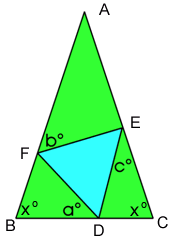#### You may also like### At a Glance

The area of a regular pentagon looks about twice as a big as the pentangle star drawn within it. Is it?### Darts and Kites

Explore the geometry of these dart and kite shapes!### No Right Angle Here

Prove that the internal angle bisectors of a triangle will never be perpendicular to each other.

# Terminology

##### Age 14 to 16Challenge Level

Correct solutions from Mary of Birchwood High School and Andrei of School 205 Bucharest have contributed to the edited solution below.

As triangle DEF is equilateral, all its angles are $60$ .Angle AEF $= 180 - 60 - c = 120 - c$

Similarly

Angle BFD $= 120 - b$
Angle EDC $= 120 - a$

From triangle FAE, I calculate angle A:

As triangle ABC is an isosceles Angle ABC $=$ Angle ACB $= x$

Therefore $180 - (120 - b + a ) = 180 - (120 - a + c )$
Therefore $60 + b - a = 60 + a - c$
Therefore $2a = b + c$
Therefore $a = (b + c)/2$

Part Two

If $a=b=c$

Angle ABC $= 180 - (120 - b + a ) = 180 - (120 - a + a ) = 60$
Angle ACB $= 180 - (120 - a + c ) = 180 - (120 - a + a ) = 60$
Therefore angle BAC is $60$

Therefore triangle ABC is equilateral.